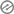# INTRODUCTION TO INDICES

FIRST TERM

Subject:

### MATHEMATICS

Term:Week:

Class:

### JSS 2 (BASIC 8)

Previous lesson:

The pupils have previous knowledge ofWHOLE NUMBERS NOTATION AND NUMERATION OF NUMBERS

that was taught as a topic during the last lesson.Topic :

INTRODUCTION TO INDICES

Behavioural objectives:

At the end of the lesson, the pupils should be able to## Instructional Materials:

• Wall charts
• Pictures
• Related Online Video
• Flash Cards

Methods of Teaching:

• Class Discussion
• Group Discussion
• Explanation
• Role Modelling
• Role Delegation

Reference Materials:• Scheme of Work
• Online Information
• Textbooks
• Workbooks
• 9 Year Basic Education Curriculum
• Workbooks

### Content

INDICES

10 × 10 × 10 = 103 is in index form, where 3 is the index or power of 103.

Similarly, p5 is short for p × p × p × p × p. 5 is the index of p in the expression p5. We often say this as ‘p to the power of 5’. The plural of index is indices.The following are the laws of indices:

Multiplication: Xa × Xb = Xa + b

Example: Multiply the following

(a) m× m5

(b) 102 × 107

(c) 5y5 × 3y3

Solutions:

(a) m× m5By expansion,

m3 × m5 = m × m × m × m × m × m × m × m = m8

m3 x m5 = m3 + 5 = m8.

(b) 102 × 107= 102 + 7 = 109

(c) 5y5 × 3y3

= (5 × 3) × y5 + 3

= 15 × y8

= 15y8Division: Xa÷ Xb = Xa – b

Example: Solve the following

(a) a7 ÷ a3

(b) 10a8 ÷ 5a6

(c) 18x5 ÷ 9x4Solution

(a) a7 ÷ a3

by expansion, we have

[Math Processing Error]

By subtracting index,a7 ÷ a3 = a7 – 3 = a4

(b) 10a8 ÷ 5a6

= (10 ÷ 5) × a8 – 6

= 2 × a2

= 2a2

(c) 18x5 ÷ 9x4

= (18 × (x5 – 4)

= 2 × x1

= 2x

Zero and negative power: Any number to the power of zero is 1 and any number having a negative power becomes a fraction. X0 = 1, X-a = 1/xa

Example: Simplify the following

(a) 10-2

(b) x5 × x-2

(c) r7× r

(d) 2a-1 × 3a2

Solution

(a) 10-2 = 1/10

(b) X5 × X-2

= X5 + (-2)= X5 – 2

= X-3

= 1/x3

(c) r7r7

=  r7-7 = r0= 1.

(d) 2a-1 × 3a2

= (2 × 3) × a-1 + 2

= 6a1

= 6a.CLASS ACTIVITY: simplify the following

(i) 2e4 × 5e10

(ii) 51m9 ÷ 3m

(iii) (3.6 × 107) ÷ (1.2 × 103)

(iv) (2a)-1 × 3a2

(v) (1/3)-2Presentation

The topic is presented step by step

Step 1:The class teacher revises the previous topics

Step 2.

He introduces the new topicStep 3:

The class teacher allows the pupils to give their own examples and he corrects them when the needs arise

### Evaluation

1. Express each of the following in standard form
• (a) 7540058
• (b) 720 000 000
• (c) 9 400 000 000
1. Express the following decimals in standard form
• (a) 05872
• (b) 0.00489
• (c) 0.000 005
1. Write the following in ordinary form
• (a)342 × 103
• (b) 9.58 × 104
1. The number 0.000 000 000 000 448 2 in standard form is
2. Simplify the following

(a) 28z12 ÷ 4z10(b) 5 x 10× 2 × 104

(c) y8 ÷ (1/y)5

Conclusion

The class teacher wraps up or concludes the lesson by giving out a short note to summarize the topic that he or she has just taught.The class teacher also goes round to make sure that the notes are well copied or well written by the pupils.

He or she makes the necessary corrections when and where the needs arise.